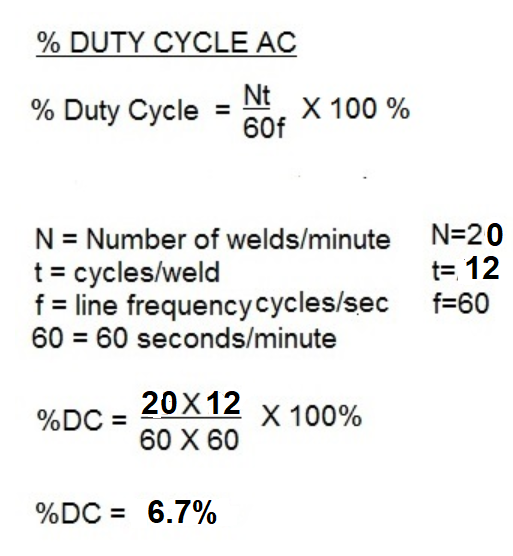# How much power is used resistance welding on a 150 KVA welder?

This question is asked often “How much power does my resistance welder use?” To answer this, we first need to calculate the Duty Cycle of the process. The formula for this is:Next one must calculate the instantaneous “KVA Demand”

The values for current can come from the control, if your control reads secondary current. If not a secondary weld analyzer compatible with resistance welding should be used.

The secondary voltage can be obtained from the transformer name plate. If there is a tap switch then use the voltage assigned to the active tap.

With this information the formula is:

KVA Instantaneous Demand = Voltage X Ka

As an Example:

Secondary Voltage – 11.5 V
Secondary Weld Current – 12.0 kA

kVA Instantaneous Demand = V x A

= 11.5V x 12kA

kVA Instantaneous Demand = 138 kVA

Next one must calculate the continuous power used by the resistance welding machine. We multiply the instantaneous demand by the square root of the duty cycle.

Using our example:

First convert the duty cycle from % to a decimal by dividing by 100.

6.67%/100 = 0.0667

Then:

kVA Continuous Demand = 138 kVA (√0.0667) = 35.64 kVA

It should be noted this value of kVA demand is actually “apparent power” (kVA).

When calculating power used or energy costs, the cost of electrical energy is in the form on Kilowatts (kW). The relationship between kVA and kW is given by the equation:

kW = kVA (cosine (PF))

where the PF is the power factor of the welding machine. The cosine of this value is typically 0.50 – 0.75. This value is dependent upon the machine design and beyond the scope of this blog. An accurate value may be obtained by a scope meter displaying the phase angle between the voltage and current. Each application will have different operating values and therefor different power consumption depending upon the job being run.
More in-depth information is available in the RWMA Resistance Welding Manual 4th Edition.

Reference: RWMA – Resistance Welding Manual 4th Edition

### Have a Question?

Do you have a question that is not covered in our knowledgebase? Do you have questions regarding the above article? Click here to ask the professor.